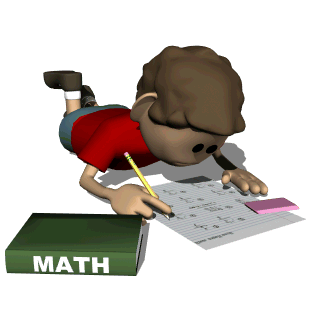Fifth grade math students will learn a variety of math topics this year including place value, numbers and operations of whole numbers, fractions, decimals, geometry and measurement. Knowledge of multiplication and division facts is essential, and students will be expected to have mastered all facts through 12s. Here are some helpful links for extra practice.

Great Valley has adopted the Math in Focus program to support our math curriculum. Please visit this webpage often to access workbook pages, tutorial videos, study materials for upcoming tests, as well as games and other resources.

Daily materials needed for math:
• Math notebook
• Red math folder
• Math textbook
• Math workbook
• Sharpened pencils
• Expo marker and eraser/old sock for whiteboards
• Assignment book